Next: 2.2 Rayleigh-Ritz Method Up: 2. Finite Element Method Previous: 2. Finite Element Method

# 2.1 The Systems of Partial Differential Equations

The general form of the PDE systems describing the physical phenomena in process and interconnect technology can be expressed through the equation system,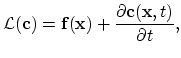(2.1)

which is defined on the simple bounded domain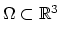. The PDE system (2.1) is fulfiled by functions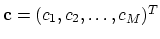,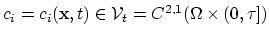, for each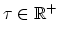. We use the notation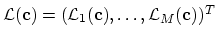,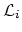is in our applications a second order nonlinear spatial differential operator and,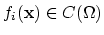.

We assume that the domain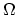has a piecewise smooth boundary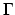. The general form of the boundary conditions applied on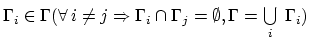,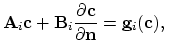defined on(2.2)

where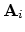and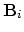are matrix consisting of functions sufficiently smooth on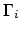and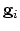is a vector of continuous linear functionals.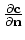denotes the outward normal derivative. The problem also demands initial conditions for each unknown function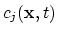,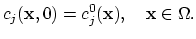(2.3)

An approximate numerical solution is sought which in some way closely resembles the exact solution. Two methods of formulating such an approximate solution are: the Rayleigh-Ritz method and Galerkin's method. In order to express the main ideas more clearly we will explain these methods for the time invariant problems in the following two sections.Next: 2.2 Rayleigh-Ritz Method Up: 2. Finite Element Method Previous: 2. Finite Element Method

H. Ceric: Numerical Techniques in Modern TCAD